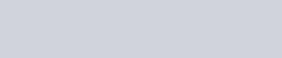A car is fitted with a convex side-view mirror of focal length 20 cm.
Question:

A car is fitted with a convex side-view mirror of focal length $20 \mathrm{~cm}$. A second car $2.8 \mathrm{~m}$ behind the first car is overtaking the first car at a relative speed of $15 \mathrm{~m} / \mathrm{s}$. The speed of the image of the second car as seen in the mirror of the first one is:-

1. $10 \mathrm{~m} / \mathrm{s}$

2. $15 \mathrm{~m} / \mathrm{s}$

3. $\frac{1}{10} \mathrm{~m} / \mathrm{s}$

4. $\frac{1}{15} \mathrm{~m} / \mathrm{s}$

Correct Option: , 4

Solution: